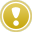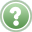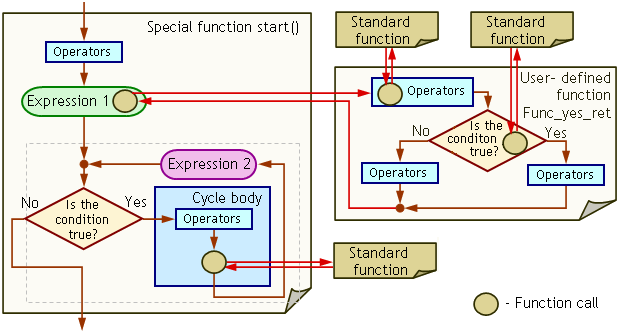English

MQL4 BookOperatorsFunction Call

# Function Call

A function call can be used as a separate operator and be found in any place in a program where it implies a certain value (with the exception of predefined cases). The format and the execution rules of a function call cover both standard (built-in) and user-defined functions.

## Function Call Format

A function call consists of the function name and the list of the passed parameters enclosed in parentheses:

`   Function_name  (Parameters_list)      // Function call as such`

The function name specified in the function call must be the same as the name of the function you want to call for execution. The parameters in the list are separated by commas. The amount of parameters to be passed to the function is limited and cannot exceed 64. In a function call, you can use constants, variables and other function calls as parameters. The amount, types and order of the passed parameters in a function call must be the same as the amount, types and order of formal parameters specified in the function description (the exception is a function call with default parameters).

`My_function (Alf, Bet)                       // Example of a function call                                             // Here:My_function                                  // Name of the called functionAlf                                          // First passed parameterBet                                          // Second passed parameter`

If the called function does not imply passing any parameters, the list of parameters is specified as empty, but the parentheses must be present, anyway.

`My_function ()                               // Exemplary function call                                             // Here:My_function                                  // Name of the called function                                             // There are no parameters to be passed`

If the program must call a function with default parameters, the list of the passed parameters can be limited (shortened). You can limit the list of parameters, starting with the first default parameter. In the example below, the local variables b, c and d have some default values:

`                                             // For the function described as:int My_function (int a, bool b=true, int c=1, double d=0.5)   {   Operators   }                                             // .. the following calls are allowed:My_function (Alf, Bet, Ham, Del)             // Allowed function callMy_function (Alf )                           // Allowed function callMy_function (3)                              // Allowed function callMy_function (Alf, 0)                         // Allowed function callMy_function (3, Tet)                         // Allowed function callMy_function (17, Bet, 3)                     // Allowed function callMy_function (17, Bet, 3, 0.5)                // Allowed function call`

The parameters without default values may not be skipped. If a default parameter is skipped, no subsequent default parameters must be specified.

`                                             // For the function described as:int My_function (int a, bool b=true, int c=1, double d=0.5)   {   Operators   }                                             // ..the following calls are ошибочными:My_function ()                               // Forbidden function call: non-default..                                             // ..parameters may not be skipped (the first one)My_function (17, Bet, , 0.5)                 // Forbidden function call: skipped..                                              // ..default parameter (the third one)`

The called functions are divided into two groups: those that return a certain value of a predefined type and those that don't return any value.

## Format of Non-Return Function Call

A call for a function that does not return any value can only be composed as a separate operator. The call function operator is ended in ";" (semicolon):

`Function_name  (Parameter_list);       // Operator of non-return function call`
`Func_no_ret (Alpha, Beta, Gamma);             // Example of a function call operator..                                             //.. for a function that does not return any value`

No other format (technique) is provided to call to functions that don't return any values.

## Format of Return Function Call

A call to a function that returns a value can be composed as a separate operator or it can be used in the program code at places where a value of a certain type is implied.

If the function call is composed as a separate operator, it ends in ";" (semicolon):

`Function_name  (Parameter_list);       // Operator of return function call`
`Func_yes_ret (Alpha, Beta, Delta);           // Example of a function call operator..                                             //.. for a function that returns a value`

## Execution Rule of Function CallA function call calls the function of the same name for execution. If the function call is composed as a separate operator, after the function has been executed, the control is passed to the operator that follows the function call. If the function call is used in an expression, after the function has been executed, the control is passed to the location in the expression, where the function call is specified; the further calculations in the expression will be performed using the value returned by the called function.

The use of function calls in other operators is determined by the format of these operators.Problem 20. Compose a program where the following conditions are realized: - if the current time is more than 15:00, execute 10 iterations in the cycle 'for'; - in all other cases, execute 6 iterations.

Below is an example of script callfunction.mq4 that includes: a function call in the header of the operator 'for' (as a part of Expression_1, according to the format of the operator 'for', see Cycle Operator 'for'), a standard function call as a separate operator, in the right part of the assignment operator (see Assignment Operator), and in the header of the operator 'if-else' (in the Condition, according to the format of the operator 'if-else', see Conditional Operator 'if-else').

`///-----------------------------------------------------------------------------------// callfunction.mq4// The code should be used for educational purpose only.//------------------------------------------------------------------------------------int start()                               // Description of function start()  {                                       // Start of the function start() body   int n;                                 // Variable declaration   int T=15;                              // Predefined time   for(int i=Func_yes_ret(T);i<=10;i++)   // The use of the function in..                                          //.the cycle operator header     {                                    // Start of the cycle 'for' body      n=n+1;                              // Iterations counter      Alert ("Iteration n=",n," i=",i);   // Function call operator     }                                    // End of the cycle 'for' body   return;                                // Exit function start()  }                                       // End of the function start() body//-------------------------------------------------------------------------------------int Func_yes_ret (int Times_in)           // Description of the user-defined function  {                                       // Start of the user-defined function body   datetime T_cur=TimeCurrent();          // The use of the function in..                                           // ..the assignment operator   if(TimeHour(T_cur) > Times_in)         // The use of the function in..                                           //..the header of the operator 'if-else'      return(1);                          // Return value 1   return(5);                             // Return value 5  }                                       // End of the user-defined function body//-------------------------------------------------------------------------------------`

In the above example, the following functions were called with the following passed parameters:

• call to the function Func_yes_ret(T) - variable T;
• call to the function Alert () - string constants "Iteration n=" and " i=", variables n and i;
• call to the function TimeCurrent() does not provide any parameters to be passed;
• call to the function TimeHour(T_cur) - variable T_cur.

A very simple algorithm is realized in this program. We set in the variable T the time (in hours), in relation to which the calculations are performed. In the header of the operator 'for', we specify the call to the user-defined function Func_yes_ret() that can return one of two values: 1 or 5. According to this value, the amount of iterations in the cycle will change: there will be either 10 (i changes from 1 to 10) or 6 (i changes from 5 to 10) iterations. For better visualization, in the cycle body we use the iteration counter, each value of which is displayed on the screen using function Alert().

In the description of the user-defined function, we first calculate the time in seconds elapsed after 00:00 of the 1st of January 1970 (call to function TimeCurrent()), and then we calculate the current time in hours (call to function TimeHour()). The algorithm is branched using the operator 'if' (call to function TimeHour() is specified in its condition). If the current time turns out to be more than that passed to the user-defined function (local variable Times_in), the latter one returns 1, otherwise it returns 5.There are no descriptions of standard functions or function call for function start() in the program.

Below you can see the block diagram of script callfunction.mq4:Fig. 51. Block diagram of a program that uses function calls.

The circles in the diagram mark function calls (for the standard and the user-defined function). Red arrows show the passing of the control into the function and vice versa. You can clearly see that the function returns the control to the location where the function call is specified, no calculations being made on the path between the function call and the function itself. Generally, if a function returns a value, this value will be passed to the calling module (along the red arrow in the direction of the function call).

Special functions can be called from any location in the program according to the general rules, on a par with other functions. Special functions can also have parameters. However, when the client terminal calls these functions, no parameters will be passed from outside, it will use the default values. The use of parameters in special functions will only be reasonable if they are called from a program. Although it is technologically possible in MQL4 to call special functions from a program, it is not recommended to do so. A program that uses special function calls should be considered as incorrect.Operator 'switch'Function Description and Operator 'return'